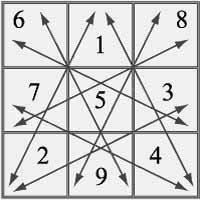Numerology

# The Psychomatrix Square and what it can revealThe Psychomatrix Square consists of a 3x3 grid, the cells of which are numbered, with each cell influencing a different trait: 1 is for character or will, 2 is for energy, 3 is for education, 4 is for health, 5 is for logic, 6 is for willingness to work, 7 is for luck, 8 is for patience and 9 is for memory. In the grid's cells, the numbers making up the date of birth are written. If any number or numbers are missing, those cells are left blank.

Along with the numbers of the date of birth, there are other special numbers written added, these are called the additional and is the main difference between the Psychomatrix Square and the Square of Pythagoras.

## How do I calculate my Psychomatrix Square?

Take December 8, 1980. Or 8/12/1980 (dd/mm/yyyy)

1. Add the digits of the day with those of the month, 8 + 1 + 2 = 11

2. Add the digits for the year, 1 + 9 + 8 + 0 = 18

3. Add the resulting numbers, 11 + 18 = 29. 29 will be the first working number.

4. Add the digits of the first working number, 29, to get the second working number, 2+9=11

5. Multiply the first digit of the date of birth (in this case, 8) by 2 and subtract from the first working number to get the third working number, 29 - 2 * 8 = 13

6. Add the the digits of the third working number, 1 + 3 = 4, to get the fourth working number.

Now you have two series of number, the first is the original date of birth, 8121980 and a string of working numbers, 2911134. After counting the digits in each series, we can begin to plot our grid.

Popular
Numerology Calculator